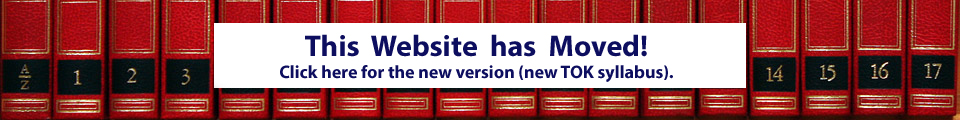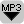## What are Formal Systems?Introduced here: the MIU puzzle as an example of a formal system. A formal system is composed of axioms, to which rules of inference are applied to produce theorems to which the rules can be applied again. Confused? Try to MIU puzzle yourself – it’s fun!

The MU Puzzle is an example of a formal system. The objective of the MU Puzzle is to try to reach the string MU starting from MI, using only these four rules:

• Rule 1: xI ? xIU. If there is an I at the end of the string of letters, then you can add a U. For example if your string is MI then you can change it into MIU. You can only add a U if the last letter is an I.
• Rule 2: Mx ? Mxx. You can double any string that follows the M. So if your string is MIU then you can double the IU after the M. You will then get MIUIU. We have doubled the IU.
• Rule 3: xIIIy ? xUy. You can replace three I in a row with one U. MUIII can be changed in to MUU. It is also possible to replace the three III if they occur in the middle somewhere. MUIIIU can also be changed to MUUU.
• Rule 4: xUUy ? xy. You can cancel and remove any two U that occur in a row. MUUU can be changed to MU.

Is it possible to reach the string MU with these four rules? If yes, then what are the steps involved? A formal system needs axioms and rules of inference. These rules are applied to the axioms and theorems are derived. It is possible to make new theorems by applying the rules to existing theorems.

The first steps in solving the the MU puzzle could be:

1. Axiom: MI
2. Theorem 1, using rule 2: MII
3. Theorem 2, using rule 2: MIIII
4. Theorem 3, using rule 3: MUI
5. Theorem 4, using rule 1: MUIU
6. Theorem 5, using rule 2: MUIUUIU
7. Theorem 6, suing rule 4: MUIIU
8. etc. until you reach MU, if possible at all.

# Transcript:

For this edition of TOK talk, you need something to write with, a pen and paper. Why? Because I’m going to show you a little puzzle for you to try out. Actually we are going through the puzzle together, but it’s difficult for you to follow by just listening to it – you need to be able to see it as well.

It’s called the MU puzzle. I want to use this puzzle as an example of a formal system. I think we’re gonna do the puzzle first and I’ll explain the theory behind formal systems later.

The puzzle starts out like this:
Write the letters MI at the top left of the page. This is our starting string of letters. And at the bottom left of the page I want you to write the letters MU. The goal of the puzzle is to change the string MI into MU. Of course you can not just cross out the I and replace it with a U. This is not allowed. There are four rules that you can use and I am going to tell you these four rules now.

I think it is a good idea to write these rules down as well. Otherwise it’s really getting kind of difficult to remember them. So, we could use the right side of the paper for writing down these rules.

Here are the four rules:

• Rule 1: If there is an I at the end of the string of letters, then you can add a U. For example if your string is MI then you can change it into MIU. You can only add a U if the last letter is an I.
• Rule 2: You can double any string that follows the M. So if your string is MIU then you can double the IU after the M. You will then get MIUIU. We have doubled the IU.
• Rule 3: You can replace three I in a row with one U. MUIII can be changed in to MUU. It is also possible to replace the three III if they occur in the middle somewhere. MUIIIU can also be changed to MUUU.
• Rule 4: You can cancel and remove any two U that occur in a row. MUUU can be changed to MU.

These are the only four rules that you can use. You do not have to use all of these rules. You can use the rules in any order that you want and of course you can use one rule as often as you want.

In summary the four rules are:

• You can add a U if there is an I at the end.
• You can double anything after the M
• You can replace III with a U
• You can remove UU

OK, let’s start. Try to write the string of letters down as we go along. I’m just going to do a couple of steps, so that you get the idea, and I’ll leave the rest up to you.

1. We have MI at the top.
2. Let’s try to double the I. This is rule 2. We can double anything that follows the M. So we get MII. We write this MII beneath the MI.
3. Why don’t we try the same thing again? Let’s double the II this time (rule 2 again). We get MIIII. That’s an M with four I. So we write this down again in the next line.
4. We could now try to replace three of these four I with one U. Which rule is that? It’s rule 3. We are allowed to replace and three I with one U. But which the Is should we exchange? We could replace the first three I or the last three I. Let’s replace the first three I with the U. We then get MUI.
5. What rule should we use next? We somehow have to eliminate this last I to get to the MU, but there is no rule that allows us to do this. We could use rule 1 again. There is an I at the end and we can add a U. So we get MUIU.
6. Slowly I’m getting frustrated, what now? Let’s try to double the UIU (rule 2). So we get MUIUUIU.
7. Ah, now there are two U in the middle! Rule 4 says that we can eliminate and two U. Let’s do that. We get MUIIU.

I hope that you get the idea. I think I’m going to stop here and I’ll leave it up to you now to try to find the solution.

Maybe you did not notice it, but you were just doing some math. The MU puzzle is something that we call a formal system, and the math that you learn in school is also a formal system. What I want to say is, that the solving of a school-math problem and the solving of the MU puzzle actually is pretty much the same thing. When you do algebra, then you apply certain rules to a mathematical formula to get a new formula. And in the MU puzzle you apply rules to a string of letters to get a new string of letters. So the last couple of minutes I was teaching you a new mathematical system. You can call it “MIU math”, if you want to. What I’m going to show you as well is that the game of chess is pretty similar to math as well.

And now to the theory part. The MU puzzle, just like algebra, is something that we call a formal system. Now what is a formal system? In a formal system you need two things.

• First of all you need a set of rules, the rules of inference, as we call them. We’ve got that. Remember the 4 rules that I told you. Algebra also has its own set of rules. And the game of chess or Go also has its own set of rules. As a matter of fact, I’m going to show you that chess is quite similar to math. And other games as well.
• The second thing that you need are so called axioms. Axioms are the starting points. The string of letters MI are the starting point for us. In chess the starting positions of the chess figures are the starting point, the axioms. These axioms can not be correct or false. They are defined and have to be accepted as they are. They are at the very beginning and have to be agreed upon. They can not be right or wrong. They are just there.

In a formal system we then apply the rules to the axioms and we do something to them. We modify the string of letters in the MU puzzle or an algebraic formula or the positions of the chess figures. We get theorems as a result. So the axioms are at the beginning, and the theorems are derived from the axioms. The rules can then also be used on the existing theorems and we get even more theorems.

These theorems can now be correct or wrong. It may quite well be possible that some of the theorems are simply not possible. You can not get there by applying the rules. In the MU puzzle the theorem UIU is not possible. There is no rule that removes the M from the string. The question is now if it is possible to arrive a the theorem MU starting from the axiom MI, and if it is possible, what rules do you have to apply in what order? In chess there are also some positions that are simply not possible with the rules of chess.

So what do we learn from that? Games such as checkers, chess, or a game of cards, are actually quite similar to math. And maybe some of you know the card game solitaire, that’s a game that you can play with yourself. You can find it on many computers. Ask yourself, what are the axioms, theorems and rules of inference of solitaire? Could it be that some games are impossible to win because of its axioms? And how are games such as solitaire different from math? Interesting, interesting……

# Questions for Discussion:

• The MU puzzle (MIU system) is a formal system, just like algebra or calculus. Why do you not learn about the MU puzzle in math class? How is the MU puzzle similar or different from school math?
• Can you come up with a list of criteria that help you determine the “value” of a formal system? You can invent pretty much any formal system that is interesting to study. Are some formal systems “more valuable” than others? What could the term “value” mean in this context?
• To what extent does intuition play a role when trying to solve the MU puzzle? Does intuition play any role at all? Or is it pure trial and error? In this context, to what extent does intuition play a role in mathematics in general?
• When learning to solve any mathematical problem, such as the MIU system, what roles do declarative and procedural knowledge play? Declarative knowledge is the knowledge of facts (“knowing that”), while procedural knowledge is the knowledge on how to do something (“knowing how”). In other words, what are the roles of facts and of practice in dealing with formal systems?